DPL 9 Help

Erlang DistributionErlang Distribution

The Erlang distribution, like the more commonly used Exponential Distribution, is a subset of the Gamma function, but with a fixed mean. Also like the Exponential Distribution, the Erlang Distribution is used to model the distribution of times between the occurrence of successive events, such as waiting times or lifetimes.

The Erlang Distribution is similar to the gamma distribution, but its mean depends on only one of its parameters. It is defined for all positive values of x. Its parameters are k and lamda, both of which must be positive. In addition, k must be an integer. The distribution is: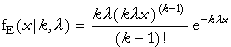where x>0, k=1,2,..., and lamda>0. Because k is an integer, the distribution includes the factorial function instead of the gamma function.

The equations for the mean and variance are: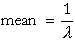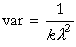The equations for the parameters are: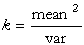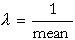The mean must be positive. Also the expression for k must produce a positive integer.

Versions: DPL Professional, DPL Enterprise, DPL Portfolio